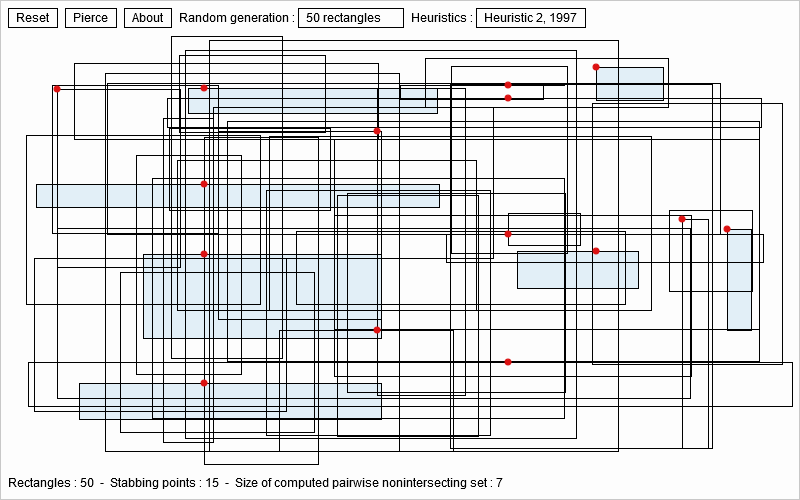# Geometric combinatorial optimization

I am interested in geometric combinatorial optimization, specially geometric Set Cover Problems (SCPs) or Hitting Set Problems (HSPs). Geometrically speaking, we want to pierce or stab a set of objects with the minimum number of points, or dually cover a set of points by a minimum number copy of a geometric shape.Caption: Piercing a set of axis-parallel rectangles with red points (Minimum Set Cover). Getting an independent set of pairwise non-intersecting axis-parallel blue rectangles (Maximum Independent Set).
Dynamic geometric combinatorial optimization problems:

Those problems are NP-hard: For example, stabbing/piercing a set of isothetic boxes or finding maximum independent set of geometric graphs. A recent breakthrough was obtained by Joseph S. B. Mitchell here (2021). I am interested in Helly, Gallai, and Hadwiger numbers as well.

Interactive demo:
Mouse left-click + drag to input rectangles. Then click on pierce button